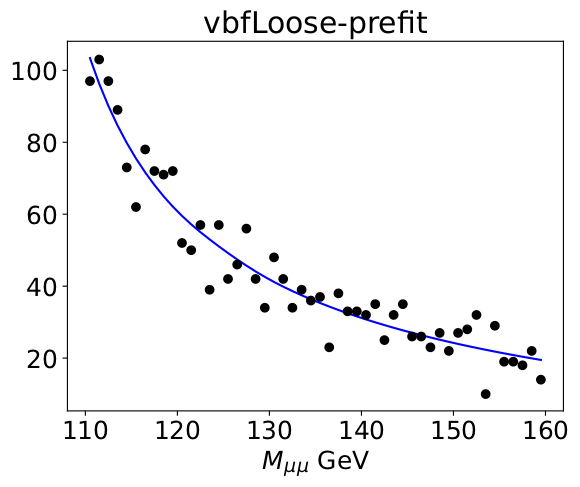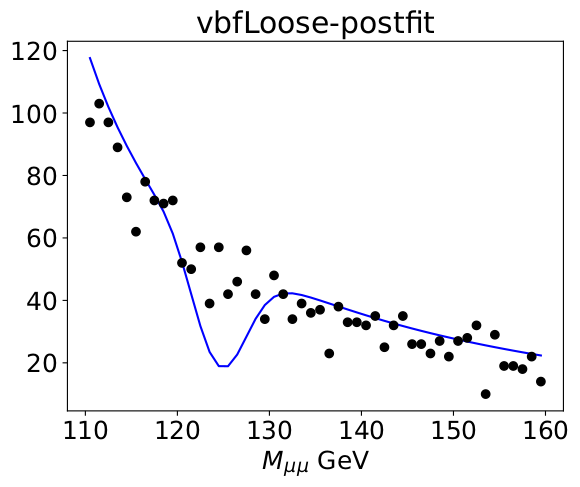# RooFit createNll gives result that is too small

Dear All,

I am performing a simultaneous fit for signal strength `mu`. When I perform a fit with `RooAbsPdf::createNll()`, I often get a result that blows up with `mu` getting too largely negative. For example, as shown in these pre-fit and post-fit plots, and a standalone example at the bottom. Could anyone help explain what might cause this? Thanks very much.

Before the fit:After the fitI have a standalone workspace with the pdf and roodatahist that I am using to make these attached.

Root file with workspace:

workspace.root (35.9 KB)

And a standalone script similar to how I am creating the NLL:

``````from __future__ import division
import sys, copy
sys.argv.append( '-b' )
from ROOT import TFile
from ROOT.RooFit import *
import ROOT
from ROOT import *

################################################################################
# standalone script for nll fit
################################################################################

# workspace
f = TFile.Open("workspace.root")
w = f.Get("w") # workspace
x = f.Get("x") # main variable
mu= f.Get("mu") # floating signal strength

# get rdh and pdf from workspace
# this is a RooDataHist with ONE "category" entry for simplicity
rdh = w.obj("simRdh")
# this is a RooSimultaneous with ONE category, and has ONE extended pdf added
pdf = w.obj("simPdf")

# create nll
nll = pdf.createNLL(rdh,RooFit.NumCPU(4))
``````

Without knowing what your Pdf actually is, it’s hard to understand whether what RooFit does actually makes sense or not

Hi Giaccaria,

Thank you for the reply. The PDF is in the attached workspace and can be viewed in a ROOT session via:

``````w->obj("simPdf")->Print()
``````

Each of the index PDFs is a sum of mu*signal + background components, something like:

``````w->obj("addPdfNonCentralHighPt")->Print()
``````

The signal model is a gaussian*CB, the background model is an exponential. The part of the code where I make the models is here:

``````            # signal model
# extend and fix range
sigPdf = RooExtendPdf(sigPdfName,sigPdfName,self.get(sigPdfName+"Pdf"),self.get(chan+"_nSig"),"bkgFitAll")
# background model
self.addStr("SUM::{0}Pdf( BW_bg{1} , frac_bg{1}[0.1,0,1] * exp_bg{1} )".format(bkgPdfName,firstUpper(chan)))_
# extend and fix range
bkgPdf = RooExtendPdf(bkgPdfName,bkgPdfName,self.get(bkgPdfName+"Pdf"),self.get(chan+"_nBkg"),"bkgFitAll")
``````

This issue is still there, if anyone has some idea what causes it any input would be helpful.
Thanks

Hi @aaronsw,

it’s hard to say after having a first look. To better understand the PDF, I used
`simPDF->graphVizTree("graph.dot")`
giving this:

Is this the PDF you wanted?

Since it’s a simPDF, I would suggest to take out categories and fit them one-by-one. You might find that one of the categories has a bizarre feature that pulls mu down, completely dominating the fits in the other categories.

Hi @StephanH,

Thanks very much for the reply. Yes, this is the PDF that I want to fit.

The example of the fits from the top post are actually made with a single category. It’s set up in the simPDF, but there’s only one addPdf and category, so all the data used in the fit is visible in those plots.

After having a second look, I wonder if the PDF does the desired thing: Isn’t there a degeneracy between mu and vbfLoose_nSig?
You extend the signal PDF with nSig, but you also multiply with the product mu * nSig, yielding mu * nSig^2. That should create a degeneracy because the coefficients are 100% anti-correlated.